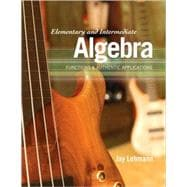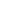Elementary and Intermediate Algebra Functions & Authentic Applications

• ISBN13:

• ISBN10:

0321621077

• Edition: 1st
• Format: Hardcover
• Publisher: Pearson

Note: Supplemental materials are not guaranteed with Rental or Used book purchases.

Purchase Benefits

•Free Shipping On Orders Over \$35!
Your order must be \$35 or more to qualify for free economy shipping. Bulk sales, PO's, Marketplace items, eBooks and apparel do not qualify for this offer.
•Get Rewarded for Ordering Your Textbooks! Enroll Now
List Price: \$223.00 Save up to \$218.89
• Rent Book
\$4.99Free Shipping

TERM
PRICE
DUE
IN STOCK USUALLY SHIPS IN 24 HOURS.
*This item is part of an exclusive publisher rental program and requires an additional convenience fee. This fee will be reflected in the shopping cart.

Supplemental Materials

What is included with this book?

• The Used and Rental copies of this book are not guaranteed to include any supplemental materials. Typically, only the book itself is included. This is true even if the title states it includes any access cards, study guides, lab manuals, CDs, etc.

Summary

Normal 0 false false false Unique in its approach, theLehmann Algebra Seriesuses curve fitting to model compelling, authentic situations, while answering the perennial question "But what is this good for?" Lehmann begins with interesting data sets, and then uses the data to find models and derive equations that fit the scenario. This interactive approach to the data helps students connect concepts and motivates them to learn. The curve-fitting approach encourages students to understand functions graphically, numerically, and symbolically. Because of the multi-faceted understanding that they gain, students are able to verbally describe the concepts related to functions. Introduction to Modeling, Operations and Expressions, Using the Slope to Graph Linear Equations, Simplifying Expressions and Solving Equations, Linear Functions and Linear Inequalities in One Variable, Systems of Linear Equations and Systems of Linear Inequalities, Polynomial Functions and Properties of Exponents, Factoring Polynomials and Solving Polynomial Equations, Quadratic Functions, Exponential Functions, Logarithmic Functions, Rational Functions, Radical Functions, Sequences and Series, Additional Topics For all readers interested in algebra.

Author Biography

In the words of the author:

Before writing my algebra series, it was painfully apparent that my students couldn't relate to the applications in the course. I was plagued with the question, "What is this good for?" To try to bridge that gap, I wrote some labs, which facilitated my students in collecting data, finding models via curve fitting, and using the models to make estimates and predictions. My students really loved working with the current, compelling, and authentic data and experiencing how mathematics truly is useful.

My students' response was so strong that I decided to write an algebra series. Little did I know that to realize this goal, I would need to embark on a 15-year challenging journey, but the rewards of hearing such excitement from students and faculty across the country has made it all worthwhile! I'm proud to have played even a small role in raising peoples' respect and enthusiasm for mathematics.

I have tried to honor my inspiration: by working with authentic data, students can experience the power of mathematics. A random-sample study at my college suggests that I am achieving this goal. The study concludes that students who used my series were more likely to feel that mathematics would be useful in their lives (P-value 0.0061) as well as their careers (P-value 0.024).

In addition to curve fitting, my approach includes other types of meaningful modeling, directed-discovery explorations, conceptual questions, and of course, a large bank of skill problems. The curve-fitting applications serve as a portal for students to see the usefulness of mathematics so that they become fully engaged in the class. Once involved, they are more receptive to all aspects of the course.

1. Introduction to Modeling

1.1 Variables and Constants

1.2 Scattergrams

1.3 Exact Linear Relationships

1.4 Approximate Linear Relationships

Taking It to the Lab

Chapter Summary

Key Points of Chapter 1

Chapter 1 Review Exercises

Chapter 1 Test

2. Operations and Expressions

2.1 Expressions

2.2 Operations with Fractions

2.4 Change in a Quantity and Subtracting Real Numbers

2.5 Ratios, Percents, and Multiplying and Dividing Real Numbers

2.6 Exponents and Order of Operations

Taking It to the Lab

Chapter Summary

Key Points of Chapter 2

Chapter 2 Review Exercises

Chapter 2 Test

Cumulative Review of Chapters 1 and 2

3. Using the Slope to Graph Linear Equations

3.1 Graphing Equations of the Form y = mx + b

3.2 Graphing Linear Models; Unit Analysis

3.3 Slope of a Line

3.4 Using the Slope to Graph Linear Equations

3.5 Rate of Change

Taking It to the Lab

Chapter Summary

Key Points of Chapter 3

Chapter 3 Review Exercises

Chapter 3 Test

4. Simplifying Expressions and Solving Equations

4.1 Commutative, Associative, and Distributive Laws

4.2 Simplifying Expressions

4.3 Solving Linear Equations in One Variable

4.4 Solving More Linear Equations in One Variable

4.5 Comparing Expressions and Equations

4.6 Formulas

Chapter Summary

Key Points of Chapter 4

Chapter 4 Review Exercises

Chapter 4 Test

Cumulative Review of Chapters 1—4

5. Linear Functions and Linear Inequalities in One Variable

5.1 Graphing Linear Equations

5.2 Functions

5.3 Function Notation

5.4 Finding Linear Equations

5.5 Finding Equations of Linear Models

5.6 Using Function Notation with Linear Models to Make Estimates and Predictions

5.7 Solving Linear Inequalities in One Variable

Taking It to the Lab

Chapter Summary

Key Points of Chapter 5

Chapter 5 Review Exercises

Chapter 5 Test

6. Systems of Linear Equations and Systems of Linear Inequalities

6.1 Using Graphs and Tables to Solve Systems

6.2 Using Substitution to Solve Systems

6.3 Using Elimination to Solve Systems

6.4 Using Systems to Model Data

6.5 Perimeter, Value, Interest, and Mixture Problems

6.6 Linear Inequalities in Two Variables; Systems of Linear Inequalities in Two Variables

Taking It to the Lab

Chapter Summary

Key Points of Chapter 6

Chapter 6 Review Exercises

Chapter 6 Test

Cumulative Review of Chapters 1—6

7. Polynomial Functions and Properties of Exponents

7.1 Adding and Subtracting Polynomial Expressions and Functions

7.2 Multiplying Polynomial Expressions and Functions

7.3 Powers of Polynomials; Product of Binomial Conjugates

7.4 Properties of Exponents

Taking It to the Lab

Chapter Summary

Key Points of Chapter 7

Chapter 7 Review Exercises

Chapter 7 Test

Making Sure You're Ready for Intermediate Algebra: A Review of Chapters 1—7

8. Factoring Polynomials and Solving Polynomial Equations

8.1 Factoring Trinomials of the Form x 2+bx+c and Differences of Two Squares

8.2 Factoring Out the GCF; Factoring by Grouping

8.3 Factoring Trinomials of the Form ax 2+bx+c

8.4 Sums and Differences of Cubes; A Factoring Strategy

8.5 Using Factoring to Solve Polynomial Equations

8.6 Using Factoring to Make Predictions with Quadratic Models

Chapter Summary

Key Points of Chapter 8

Chapter 8 Review Exercises

Chapter 8 Test

Making Sure You're Ready for Intermediate Algebra: A Review of Chapters 1—8

9.1 Graphing Quadratic Functions in Vertex Form

9.2 Graphing Quadratic Functions in Standard Form

9.4 Using the Square Root Property to Solve Quadratic Equations

9.5 Solving Quadratic Equations by Completing the Square

9.7 Solving Systems of Linear Equations in Three Variables; Finding Quadratic Functions

Taking It to the Lab

Chapter Summary

Key Points of Chapter 9

Chapter 9 Review Exercises

Chapter 9 Test

Cumulative Review of Chapters 1—9

10. Exponential Functions

10.1 Integer Exponents

10.2 Rational Exponents

10.3 Graphing Exponential Functions

10.4 Finding Equations of Exponential Functions

10.5 Modeling with Exponential Functions

Taking It to the Lab

Chapter Summary

Key Points of Chapter 10

Chapter 10 Review Exercises

Chapter 10 Test

11. Logarithmic Functions

11.1 Inverse Functions

11.2 Logarithmic Functions

11.3 Properties of Logarithms

11.4 Using the Power Property with Exponential Models to Make Predictions

11.5 More Properties of Logarithms

11.6 Natural Logarithms

Taking It to the Lab

Chapter Summary

Key Points of Chapter 11

Chapter 11 Review Exercises

Chapter 11 Test

Cumulative Review of Chapters 1—11

12. Rational Functions

12.1 Finding the Domains of Rational Functions and Simplifying Rational Expressions

12.2 Multiplying and Dividing Rational Expressions

12.3 Adding and Subtracting Rational Expressions

12.4 Simplifying Complex Rational Expressions

12.5 Solving Rational Equations

12.6 Modeling with Rational Functions

12.7 Proportions; Similar Triangles

12.8 Variation

Taking It to the Lab

Chapter Summary

Key Points of Chapter 12

Chapter 12 Review Exercises

Chapter 12 Test

13.3 Rationalizing Denominators and Simplifying Quotients of Radical Expressions

13.4 Graphing and Combining Square Root Functions

13.6 Modeling with Square Root Functions

Taking It to the Lab

Chapter Summary

Key Points of Chapter 13

Chapter 13 Review Exercises

Chapter 13 Test

14. Sequences and Series

14.1 Arithmetic Sequences

14.2 Geometric Sequences

14.3 Arithmetic Series

14.4 Geometric Series

Taking It to the Lab

Chapter Summary

Key Points of Chapter 14

Chapter 14 Review Exercises

Chapter 14 Test

Cumulative Review of Chapters 1—14

15.1 Absolute Value: Equations and Inequalities

15.2 Performing Operations with Complex Numbers

15.3 Pythagorean Theorem, Distance Formula, and Circles

15.4 Ellipses and Hyperbolas

15.5 Solving Nonlinear Systems of Equations

Appendix A: Using a TI-83/84 Graphing Calculator